# Create a scatter plot using pandas DataFrame (`pandas.DataFrame.plot.scatter`)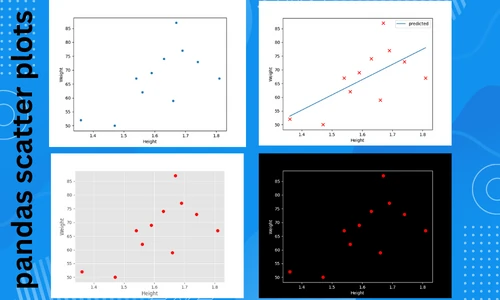The scatter plot visualizes the relationship between two or three numerical variables by plotting individual data points. A pairwise scatter plot can be created if you have more than three variables. Scatter plots are useful for visualizing correlation, clustering, regression, and classification analyses.

In Python, scatter plots are typically created from numerical arrays using the `pyplot.scatter()` function available in the matplotlib package. The scatter plots in Pandas, however, allow you to plot directly using columns from the Dataframe. This tutorial will show you how to create a scatter plot directly from a pandas DataFrame using a `plot.scatter()` function.

The `plot.scatter()` function takes the following arguments for creating a scatter plot,

• x: column name for plotting data on X-axis
• y: column name for plotting data on Y-axis
• s: the size of the points. It can take a single scalar value or a numerical array
• c: the color of the points (RGB or RGBA code). It can take a single scalar value or multiple values

Now, let’s create a scatter plot using pandas `plot.scatter()`. We will use students height and weight data for creating a scatter plot. The height and weight variables will be plotted on the X and Y-axis, respectively.

## Import the pandas library

Import the pandas library

``````import pandas as pd
``````

## Import the dataset

Import the dataset as pandas DataFrame

``````df = pd.read_csv('https://reneshbedre.github.io/assets/posts/reg/height.csv')
# view first two rows of dataset
# output
Height  Weight
0    1.36      52
1    1.47      50
``````

## Create a scatter plot

Create a basic scatter plot (with default shape and color),

``````df.plot.scatter(x = 'Height', y = 'Weight')
plt.show()
``````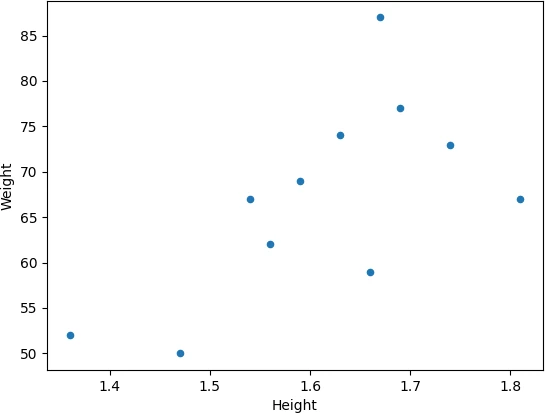Change the size (`s`) and colors (`c`) of the points,

``````df.plot.scatter(x = 'Height', y = 'Weight', s = 50, c = 'red')
plt.show()
``````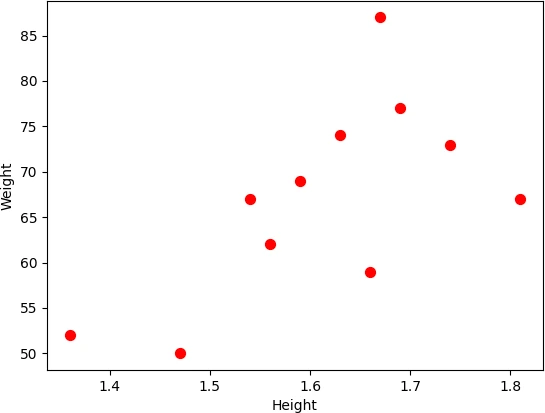Change marker shape (`marker`) and transparency (`alpha`),

``````df.plot.scatter(x = 'Height', y = 'Weight', s = 100, marker = 'x', alpha = 0.5)
plt.show()
``````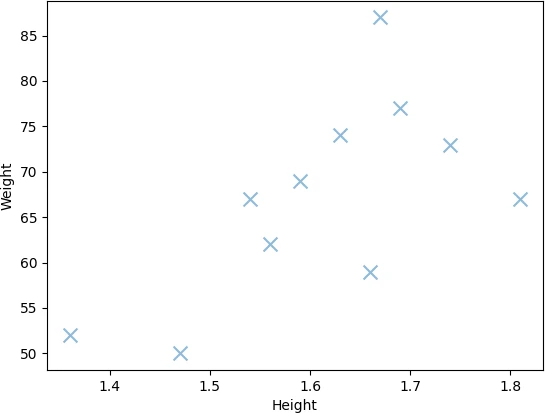Add a title (`title`) to pandas scatter plot,

``````df.plot.scatter(x = 'Height', y = 'Weight', s = 50, title = 'pandas scatter plot')
plt.show()
``````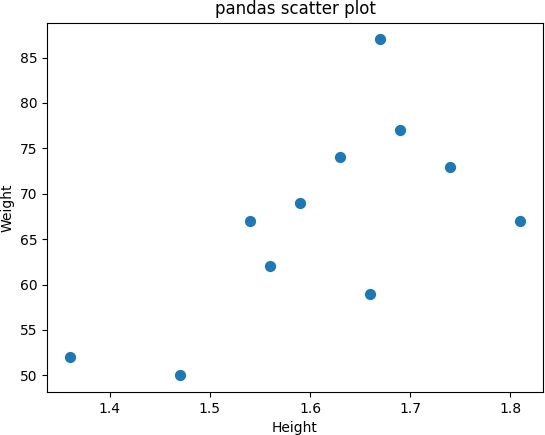Add legend to pandas scatter plot using `plt.legend()` function. You can specify the location of the legend using the `loc` parameter,

``````df.plot.scatter(x = 'Height', y = 'Weight', s = 50, title = 'pandas scatter plot', label = 'scatter')
plt.legend(loc = 'upper left')
plt.show()
``````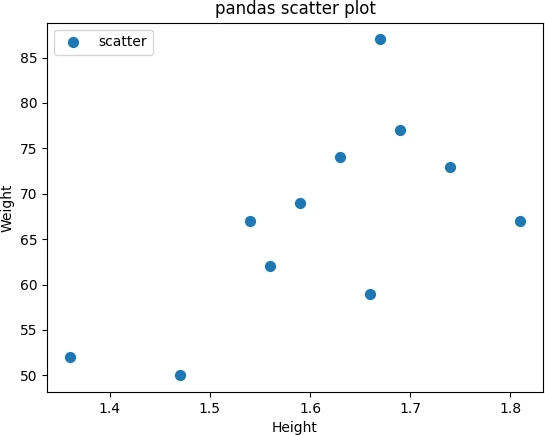Add X and Y-axis labels to the pandas scatter plot using `plt.xlabel()` and `plt.ylabel()` functions,

``````df.plot.scatter(x = 'Height', y = 'Weight', s = 50, title = 'pandas scatter plot', label = 'scatter')
plt.xlabel('Height (m)')
plt.ylabel('Weight (kg)')
plt.show()
``````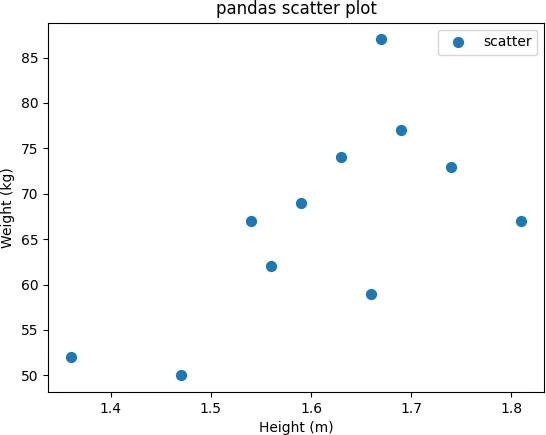Add X and Y-axis limits to the pandas scatter plot using `xlim` and `ylim` functions. You can specify the left and right range (as shown in code below).

``````df.plot.scatter(x = 'Height', y = 'Weight', s = 50,  marker = 'x', c = '#030764', title = 'pandas scatter plot')
plt.xlim(1, 2)
plt.ylim(40, 90)
plt.show()
``````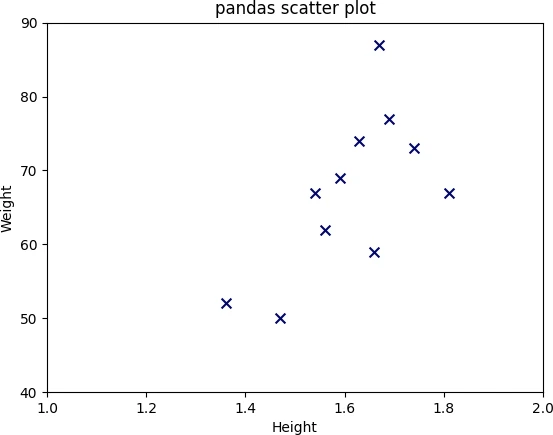You can also change the style of the pandas scatter plot using `plt.style.use()` function. You can either change the background color or the theme of the plot. For example, create a dark background and ggplot style scatter plot,

``````# dark background
plt.style.use('dark_background')

df.plot.scatter(x = 'Height', y = 'Weight', s = 50, c = 'red')
plt.show()

# ggplot style
plt.style.use('ggplot')

df.plot.scatter(x = 'Height', y = 'Weight', s = 50, c = 'red')
plt.show()
``````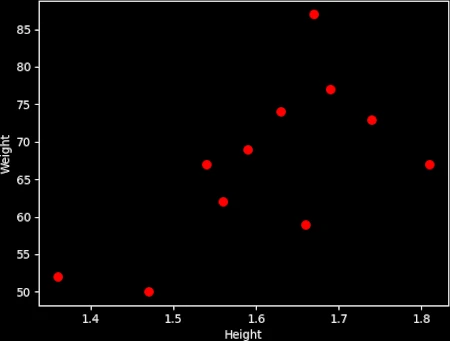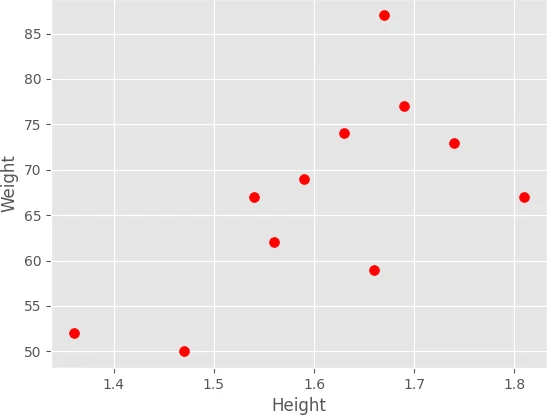If you want switch back to default plotting style, you can use `plt.style.use('default')` function.

Add a regression line on the scatter plot. First, to get a regression line, we will fit the model using the sklearn `LinearRegression()` function and then plot a fitted line on a scatter plot.

``````from sklearn.linear_model import LinearRegression
import numpy as np

X = np.array(df['Height']).reshape(-1, 1)
y = np.array(df['Weight'])
# fit the model
reg = LinearRegression().fit(X, y)
df['predicted'] = reg.predict(X)

fig, ax = plt.subplots()
df.plot.scatter(x = 'Height', y = 'Weight', s = 50,  marker = 'x', c = 'red', ax = ax)
df.plot.line(x = 'Height', y = 'predicted', ax = ax)
plt.show()
``````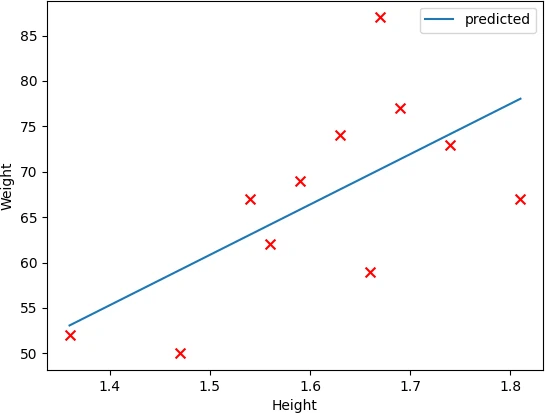In summary, you learned how to create a pandas scatter plot on DataFrame using pandas `plot.scatter()` function. You can learn how to create a line plot in Pandas by reading this article.